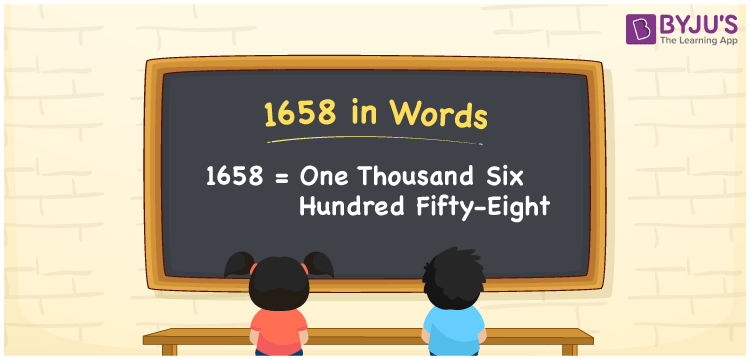# 1658 in Words

We can write 1658 in words as One thousand six hundred fifty-eight. If you spent Rs. 1658 for a face pack, then you can say, “I spent One thousand six hundred fifty-eight rupees for a face pack”. From this, we can say that the cardinal number 1658 is written in word form as “One thousand six hundred fifty-eight”.

 1658 in words One thousand six hundred fifty-eight One thousand six hundred fifty-eight in Numbers 1658

## 1658 in English Words

We generally write numbers in words using the English alphabet. So, we spell 1658 in English as “One thousand six hundred fifty-eight”.## How to Write 1658 in Words?

The below table is defined as the place value chart for the number 1658. From this table, we can convert 1658 into words.

 Thousands Hundreds Tens Ones 1 6 5 8

Here, ones = 8, tens = 5, hundreds = 6, and thousands = 1.

The above numbers can be expanded as,

1 × Thousand + 6 × Hundred + 5 × Ten + 8 × One

= 1 × 1000 + 6 × 100 + 5 × 10 + 8 × 1

= 1000 + 600 + 50 + 8

= One thousand + Six hundred + Fifty + Eight

= One thousand six hundred fifty-eight

Therefore, 1658 in words = One thousand six hundred fifty-eight.

As we know, 1658 is a natural number that precedes 1659 and succeeds 1657.

1658 in words – One thousand six hundred fifty-eight

Is 1658 an odd number? – No

Is 1658 an even number? – Yes

Is 1658 a prime number? – No

Is 1658 a composite number? – Yes

Is 1658 a perfect square number? – No

Is 1658 a perfect cube number? – No

## Frequently Asked Questions on 1658 in Words

Q1

### How do you write 1658 in words?

We can write 1658 in words as One thousand six hundred fifty-eight.
Q2

### How to write Rs. 1658 in words on a cheque?

On a cheque, we generally write Rs. 1658 in words as “One thousand six hundred fifty-eight rupees only”.
Q3

### What is 1658 in word form?

One thousand six hundred fifty-eight denotes the number 1658 in word form.